# Stochastic Optimal Economic Growth Model¶

Welfare maximizing social planner must decide how much society should consume and invest. Unlike the deterministic model, this model allows arbitrary constant relative risk aversion, capital depreciation, and stochastic production shocks. It lacks a known closed-form solution.

• States
• s stock of wealth
• Actions
• k capital investment
• Parameters
• alpha relative risk aversion
• beta capital production elasticity
• gammma capital survival rate
• sigma production shock volatility
• delta discount factor
In :
import numpy as np
import matplotlib.pyplot as plt
from compecon import BasisChebyshev, DPmodel, DPoptions, qnwlogn, demo
import seaborn as sns
import pandas as pd


### Model parameters¶

In :
α, β, γ, σ, δ = 0.2, 0.5, 0.9, 0.1, 0.9


## Analytic results¶

The deterministic steady-state values for this model are

In :
kstar = ((1 - δ*γ)/(δ*β))**(1/(β-1))  # steady-state capital investment

sstar = γ*kstar + kstar**β            # steady-state wealth


## Numeric results¶

### State space¶

The state variable is s="Wealth", which we restrict to $s\in[5, 10]$.

Here, we represent it with a Chebyshev basis, with $n=10$ nodes.

In :
n, smin, smax = 10, 5, 10
basis = BasisChebyshev(n, smin, smax, labels=['Wealth'])


### Continuous state shock distribution¶

In :
m = 5
e, w = qnwlogn(m, -σ**2/2,σ**2)


### Action space¶

The choice variable k="Investment" must be nonnegative.

In :
def bounds(s, i=None, j=None):
return np.zeros_like(s), 0.99*s


### Reward function¶

The reward function is the utility of consumption=$s-k$.

In :
def reward(s, k, i=None, j=None):
sk = s - k
u = sk**(1-α) / (1-α)
ux= -sk **(-α)
uxx = -α * sk**(-1-α)
return u, ux, uxx


### State transition function¶

Next period, wealth will be equal to production from available initial capital $k$, that is $s' = k^\beta$

In :
def transition(s, k, i=None, j=None, in_=None, e=None):
g = γ * k  + e * k**β
gx = γ  + β*e * k**(β-1)
gxx = β*(β-1)*e * k**(β-2)
return g, gx, gxx


### Model structure¶

The value of wealth $s$ satisfies the Bellman equation \begin{equation*} V(s) = \max_k\left\{\log(s-k) + \delta V(k^\beta) \right\} \end{equation*}

To solve and simulate this model,use the CompEcon class DPmodel

In :
growth_model = DPmodel(basis, reward, transition, bounds,e=e,w=w,
x=['Investment'],
discount=δ )


### Solving the model¶

Solving the growth model by collocation.

In :
S = growth_model.solve()

Solving infinite-horizon model collocation equation by Newton's method
iter change       time
------------------------------
0       8.2e+00    0.0035
1       9.6e+00    0.1350
2       2.2e+00    0.2040
3       3.8e-02    0.2666
4       7.0e-06    0.3038
5       2.0e-14    0.3212
Elapsed Time =    0.32 Seconds


DPmodel.solve returns a pandas DataFrame with the following data:

We are also interested in the shadow price of wealth (the first derivative of the value function).

To analyze the dynamics of the model, it also helps to compute the optimal change of wealth.

In :
S['shadow price'] = growth_model.Value(S['Wealth'],1)
S['D.Wealth'] = transition(S['Wealth'], S['Investment'],e=1) - S['Wealth']

Out:
Wealth value resid Investment shadow price D.Wealth
Wealth
5.000000 5.000000 17.793592 4.324111e-09 3.997670 0.999535 0.597321
5.050505 5.050505 17.843995 -1.837414e-09 4.032514 0.996440 0.586869
5.101010 5.101010 17.894243 -4.078480e-09 4.067300 0.993391 0.576314
5.151515 5.151515 17.944339 -3.954337e-09 4.102028 0.990386 0.565657
5.202020 5.202020 17.994283 -2.583509e-09 4.136700 0.987425 0.554898

### Solving the model by Linear-Quadratic Approximation¶

The DPmodel.lqapprox solves the linear-quadratic approximation, in this case arround the steady-state. It returns a LQmodel which works similar to the DPmodel object. We also compute the shadow price and the approximation error to compare these results to the collocation results.

In :
growth_lq = growth_model.lqapprox(sstar, kstar)
L = growth_lq.solution(basis.nodes)
L['D.Wealth'] = L['Wealth_next']- L['Wealth']

Out:
Wealth Investment value value_Wealth Wealth_next shadow price D.Wealth
Wealth
5.030779 5.030779 3.461913 17.923286 0.911808 5.030781 0.911808 1.397911e-06
5.272484 5.272484 3.679447 18.143387 0.909432 5.272485 0.909432 1.256542e-06
5.732233 5.732233 4.093221 18.560459 0.904912 5.732234 0.904912 9.876423e-07
6.365024 6.365024 4.662732 19.131111 0.898692 6.365024 0.898692 6.175332e-07
7.108914 7.108914 5.332233 19.796920 0.891380 7.108914 0.891380 1.824439e-07

## Plotting the results¶

### Optimal Policy¶

In :
fig1 = demo.figure('Optimal Investment Policy', 'Wealth', 'Investment')
plt.plot(S.Investment, label='Chebychev Collocation')
plt.plot(L.Investment, label='L-Q Approximation')
demo.annotate(sstar, kstar,'$s^*$ = %.2f\n$V^*$ = %.2f' % (sstar, kstar),'bo', (10, -17),ms=10)
plt.legend(loc= 'upper left')

Out:
<matplotlib.legend.Legend at 0x1949a3e9be0>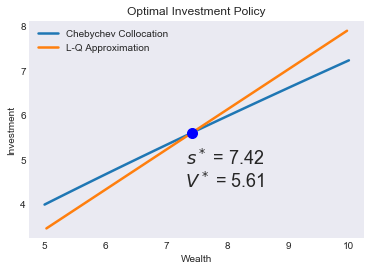### Value Function¶

In :
fig2 = demo.figure('Value Function', 'Wealth', 'Value')
plt.plot(S.value, label='Chebychev Collocation')
plt.plot(L.value, label='L-Q Approximation')
plt.legend(loc= 'upper left')

Out:
<matplotlib.legend.Legend at 0x1949a7a7da0>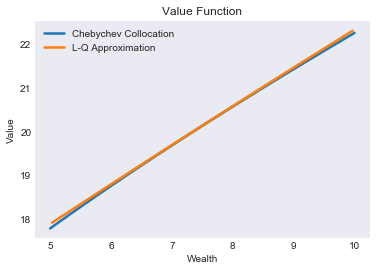In :
fig3 = demo.figure('Shadow Price Function', 'Wealth', 'Shadow Price')
plt.legend(loc= 'upper right')

Out:
<matplotlib.legend.Legend at 0x1949a9af4a8>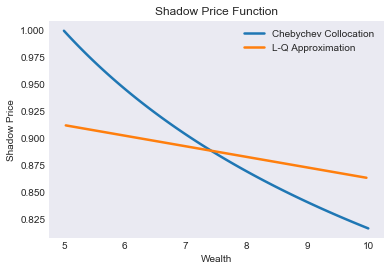### Chebychev Collocation Residual¶

In :
fig4 = demo.figure('Bellman Equation Residual', 'Wealth', 'Residual')
plt.hlines(0,smin,smax,'k',linestyles='--')
plt.plot(S[['resid']])
plt.ticklabel_format(style='sci', axis='y', scilimits=(-1,1))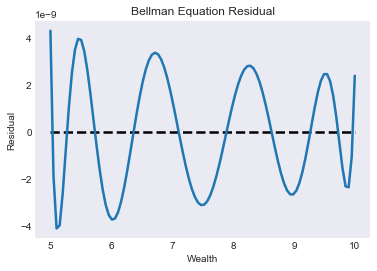### Wealth dynamics¶

Notice how the steady-state is stable in the Chebyshev collocation solution, but unstable in the linear-quadratic approximation. In particular, simulated paths of wealth in the L-Q approximation will converge to zero, unless the initial states is within a small neighborhood of the steady-state.

In :
fig5 = demo.figure('Wealth dynamics', 'Wealth', 'Wealth change', figsize=[8,5])
plt.plot(S['D.Wealth'], label='Chebychev Collocation')
plt.plot(L['D.Wealth'], label='L-Q Approximation')
plt.hlines(0,smin,smax,linestyles=':')

demo.annotate(sstar, 0, f'$s^* = {sstar:.2f}$\n$\Delta s^* = {0:.2f}$', 'bo', (10, 10),ms=10,fs=11)
plt.legend(loc= 'lower left')

Out:
<matplotlib.legend.Legend at 0x1949aa4bc18>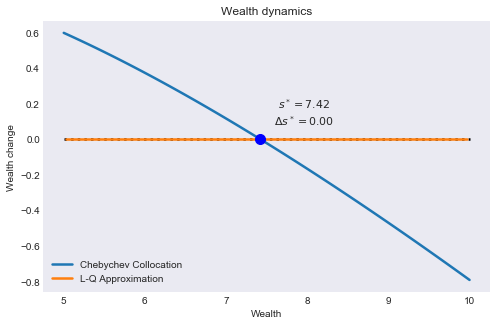### Simulating the model¶

We simulate 21 periods of the model starting from $s=s_{\min}$

In :
T = 21
nrep = 50_000
data = growth_model.simulate(T, np.tile(smin,nrep))


### Simulated State and Policy Paths¶

In :
subdata = data[data['_rep'].isin(range(3))]
opts = dict(spec='r*', offset=(0, -15), fs=11, ha='right')

In :
fig6 = demo.figure('Simulated and Expected Wealth','Period', 'Wealth',[0, T + 0.5])
plt.plot(data[['time','Wealth']].groupby('time').mean())
plt.plot(subdata.pivot('time','_rep','Wealth'),lw=1)
demo.annotate(T, sstar, f'steady-state wealth\n = {sstar:.2f}', **opts)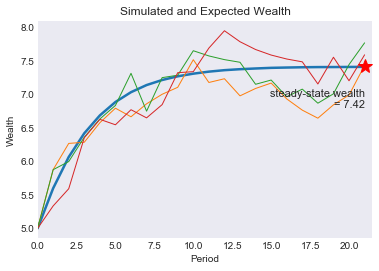In :
fig7 = demo.figure('Simulated and Expected Investment','Period', 'Investment',[0, T + 0.5])
plt.plot(data[['time','Investment']].groupby('time').mean())
plt.plot(subdata.pivot('time','_rep','Investment'),lw=1)
demo.annotate(T, kstar, f'steady-state investment\n = {kstar:.2f}', **opts)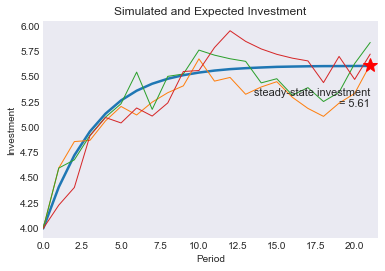### Ergodic Wealth Distribution¶

In :
subdata = data[data['time']==T][['Wealth','Investment']]
'Ergodic Means': subdata.mean(),
'Ergodic Standard Deviations': subdata.std()}).T
stats

Out:
Wealth Investment
fig8 = demo.figure('Ergodic Wealth and Investment Distribution','Wealth','Probability',[4.5, 9.5])

<matplotlib.axes._subplots.AxesSubplot at 0x1949aba3390>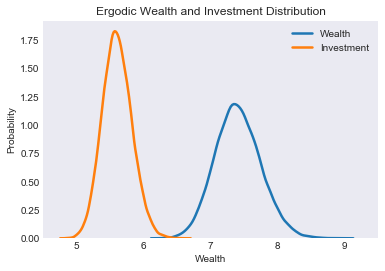#demo.savefig([fig1,fig2,fig3,fig4,fig5,fig6,fig7,fig8])